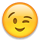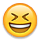## Forum Stats

• 3,733,247 Users
• 2,246,737 Discussions
• 7,856,631 Comments

Discussions

#### Howdy, Stranger!

It looks like you're new here. If you want to get involved, click one of these buttons!

# Get z record fast (from x to y records)

Hello gurus,

I have table a containing around 7 milion records and trying to retrieve z record.
I have tried to this script

SELECT id, name
FROM
(
SELECT ROWNUM rn, id, name
FROM table_a
ORDER BY name
)
WHERE rn BETWEEN 5 to 10;

Already use index for the table and it retrieve fast if I start from rownum = 1.
And have tried using hints FIRST_ROWS but doesnt do any good.
Is there any way to make it faster?

Thank you so much
henry

## Comments

• You're ordering 7 million rows, which means every record is touched.

I think you need to be a bit more explicit with the actual requirements, is this a paging of results for a web gui?
• You should not use between

Look at this:
```SQL> create table t1 as select object_name, object_id from all_objects;
Table created.
SQL> select count(*) from t1;
COUNT(*)
--------------------
4131
SQL> exec dbms_stats.gather_table_stats( user, 'T1');
PL/SQL procedure successfully completed.First we use the standard query for the "top-n" records
SQL> get tt
1  select *
2  from       ( select t1.*, rownum rn
3       from   t1
4       where  rownum < 12
5       order by object_name
6     ) v1
7* where      v1.rn > 8
SQL> /
OBJECT_NAME                               OBJECT_ID                   RN
------------------------------ -------------------- --------------------
STANDARD                                        309                    9
V\$DLM_LATCH                                     314                   11
V\$DLM_MISC                                      312                   10
Execution Plan
----------------------------------------------------------
0      SELECT STATEMENT Optimizer=CHOOSE (Cost=4 Card=11 Bytes=473)
1    0   VIEW (Cost=4 Card=11 Bytes=473)
2    1     SORT (ORDER BY) (Cost=4 Card=11 Bytes=242)
3    2       COUNT (STOPKEY)
4    3         TABLE ACCESS (FULL) OF 'T1' (TABLE) (Cost=2 Card=4131 Bytes=90882)Can you see how the optimizer had only to select the first 11 records (out from 4131 reords)
SQL> get tt
1  select *
2  from       ( select t1.*, rownum rn
3       from   t1
4       where  rownum < 1012
5       order by object_name
6     ) v1
7* where      v1.rn > 1008
SQL> /
OBJECT_NAME                               OBJECT_ID                   RN
------------------------------ -------------------- --------------------
DBA_INDEXTYPES                                22209                 1009
USER_INDEXTYPES                               22210                 1010
USER_INDEXTYPES                               22211                 1011
Execution Plan
----------------------------------------------------------
0      SELECT STATEMENT Optimizer=CHOOSE (Cost=4 Card=1011 Bytes=43473)
1    0   VIEW (Cost=4 Card=1011 Bytes=43473)
2    1     SORT (ORDER BY) (Cost=4 Card=1011 Bytes=22242)
3    2       COUNT (STOPKEY)
4    3         TABLE ACCESS (FULL) OF 'T1' (TABLE) (Cost=2 Card=4131 Bytes=90882)Can you see how the optimizer had only to select the first 1011 records (out from 4131 reords)Now we use your between for "top-n" records
SQL> get ttt
1  select *
2  from (
3         select t1.*, rownum rn
4         from t1
5         order by object_name
6       )
7* where rn between 9 and 11
SQL> /
OBJECT_NAME                               OBJECT_ID                   RN
------------------------------ -------------------- --------------------
STANDARD                                        309                    9
V\$DLM_LATCH                                     314                   11
V\$DLM_MISC                                      312                   10
Execution Plan
----------------------------------------------------------
0      SELECT STATEMENT Optimizer=CHOOSE (Cost=4 Card=4131 Bytes=177633)
1    0   VIEW (Cost=4 Card=4131 Bytes=177633)
2    1     SORT (ORDER BY) (Cost=4 Card=4131 Bytes=90882)
3    2       COUNT
4    3         TABLE ACCESS (FULL) OF 'T1' (TABLE) (Cost=2 Card=4131 Bytes=90882)Can you see how the optimizer had to select all 4131 records (even though you only wanted to see the first 11 records
SQL> get ttt
1  select *
2  from (
3         select t1.*, rownum rn
4         from t1
5         order by object_name
6       )
7* where rn between 1009 and 1011
SQL> /
OBJECT_NAME                               OBJECT_ID                   RN
------------------------------ -------------------- --------------------
DBA_INDEXTYPES                                22209                 1009
USER_INDEXTYPES                               22210                 1010
USER_INDEXTYPES                               22211                 1011
Execution Plan
----------------------------------------------------------
0      SELECT STATEMENT Optimizer=CHOOSE (Cost=4 Card=4131 Bytes=177633)
1    0   VIEW (Cost=4 Card=4131 Bytes=177633)
2    1     SORT (ORDER BY) (Cost=4 Card=4131 Bytes=90882)
3    2       COUNT
4    3         TABLE ACCESS (FULL) OF 'T1' (TABLE) (Cost=2 Card=4131 Bytes=90882)Can you see how the optimizer had again to select all 4131 records
SQL> ```
• b]First we use the standard query for the "top-n"
records
SQL> get tt
1 select *
2 from ( select t1.*, rownum rn
3 from t1 > 4 where rownum < 12 5 order by object_name6 ) v1
7* where v1.rn > 8
L> /
Is it really the standard top-n query ?
Take a look here : On Top-n and Pagination Queries

Nicolas.
• You got me, Nicolas ;-)
I had included the order by without rewriting the query. Nevertheless between is a lot slower (and it is not even giving a correct result with the order by) than the other construct.
```SQL> select count(*) from t1;
COUNT(*)
--------------------
57834
SQL> set autotrace on
SQL> get tt
1  select *
2  from       ( select v1.*, rownum rn
3       from   (
4                     select t1.*
5                     from   t1
6                     order by object_name
7              ) v1
8       where  rownum < 12
9     ) v2
10* where      v2.rn > 8
SQL> /
OBJECT_ID OBJECT_NAME                                      RN
-------------------- ------------------------------ --------------------
957651 AGGXMLIMP                                         9
957651 AGGXMLIMP                                        10
957651 AGGXMLIMP                                        11
Execution Plan
----------------------------------------------------------
0      SELECT STATEMENT Optimizer=CHOOSE (Cost=229 Card=11 Bytes=473)
1    0   VIEW (Cost=229 Card=11 Bytes=473)
2    1     COUNT (STOPKEY)
3    2       VIEW (Cost=229 Card=58581 Bytes=1757430)
4    3         SORT (ORDER BY STOPKEY) (Cost=229 Card=58581 Bytes=1288782)
5    4           TABLE ACCESS (FULL) OF 'T1' (TABLE) (Cost=12 Card=58581 Bytes=1288782)
Statistics
----------------------------------------------------------
0  recursive calls
0  db block gets
112  consistent gets
0  physical reads
0  redo size
740  bytes sent via SQL*Net to client
660  bytes received via SQL*Net from client
2  SQL*Net roundtrips to/from client
1  sorts (memory)
0  sorts (disk)
3  rows processed
SQL> get ttt
1  select *
2  from (
3         select t1.*, rownum rn
4         from t1
5         order by object_name
6       )
7* where rn between 9 and 11
SQL> /
OBJECT_ID OBJECT_NAME                                      RN
-------------------- ------------------------------ --------------------
282388 GEN_AUDIT_GEN                                    11
113460 PRS_OEHIERARCHIE                                 10
1531875 SQLN_EXPLAIN_PLAN                                 9
Execution Plan
----------------------------------------------------------
0      SELECT STATEMENT Optimizer=CHOOSE (Cost=229 Card=58581 Bytes=2518983)
1    0   VIEW (Cost=229 Card=58581 Bytes=2518983)
2    1     SORT (ORDER BY) (Cost=229 Card=58581 Bytes=1288782)
3    2       COUNT
4    3         TABLE ACCESS (FULL) OF 'T1' (TABLE) (Cost=12 Card=58581 Bytes=1288782)
Statistics
----------------------------------------------------------
1  recursive calls
8  db block gets
112  consistent gets130 physical reads
0  redo size
790  bytes sent via SQL*Net to client
660  bytes received via SQL*Net from client
2  SQL*Net roundtrips to/from client
0  sorts (memory)
1  sorts (disk)
3  rows processed
SQL>
[pre]
Message was edited by:
Leo Mannhart                                                                                                                                                                                                                                                                                                                                                                                                                                                                                                                                                                                                                                                                                                                                                                                                                                                                                                                                                                                                                                                                                                                                                                                                                                                                                                                                                                                                                                                                                                                                                                                                                                                                                                                                                                                                                                                                                                                                                                                                                                                                                                                                                                                                                                                                                                                                                                                                                                                                                                                                                                                                                                                                                                                                                                                                                                                                                                                                                                                                                                                                                                                                                                                                                                                                                                                                                                                                                                                                                                                                                                                                                                                                                                                                                                                                                                                                                                                                                                                                                                                                                                                                                                                                                                                                                                                                                                                                                                                                                                                                                                                                                                                                                                                                                                                                                                                                                                                                                                                                                                                                                                                                                                                                                                                                                                                                                                                                                                                                                                                                                                                                                                                                                                                                                                                                                                                                                                                                                                                                                                                                                                                                                                                                                                                                                                                                                                                                                                                                                                                                                                                                                                                                                                                                                                                                                                                                                                                                                                                                                                                                                                                                                                                                                        ```
• 1 select *
2 from (
3 select t1.*, rownum rn
4 from t1
5 order by object_name
6 )
7* where rn between 9 and 11
It would still not be the right comparison. You need to use
```select *
from (select t.*, rownum rn
from (select   *
from t1
order by object_name) t1)
where rn between 9 and 11```• If ranks are unique,
we can use sometimes fast version of Pagination in Getting Rows N Through M.

If ranks are unique,
we can avoid full Scan.

These solutions are using Count STOPKEY.
Because we can derive maxSelectedRecordCount using "maxSelectedRecordCount = EndRank-StartRank+1".

Then,
If we know maxSelectedRecordCount,
we can use RowNum <= maxSelectedRecordCount
```create table TestTable(
SortKey    number primary key,
anyColumns number);
begin
for i in 1..1000/*or more large number*/ Loop
insert into TestTable values(i*2,i*5);
commit;
end Loop;
end;
/```
for instance (StartRank is 100 and EndRank is 200.)
```--Using subquery
select SortKey,anyColumns
from TestTable a
where (select count(b.SortKey)+1
from TestTable b
where b.SortKey <= a.SortKey
and RowNum <= 200) between 100 and 200
and RowNum <= (200-100)+1
order by SortKey desc;```
```--Sort in InlineView
select SortKey,anyColumns
from (select SortKey,anyColumns,RowNum as Rank
from (select SortKey,anyColumns from TestTable order by SortKey desc))
where Rank between 100 and 200
and RowNum <= (200-100)+1
order by SortKey desc;```
```--Using OLAP
select SortKey,anyColumns
from (select SortKey,anyColumns,Row_Number() over(order by SortKey desc) as Rank
from TestTable)
where Rank between 100 and 200
and RowNum <= (200-100)+1
order by SortKey desc;```
• Got me again ;-)

But it doesn't change the picture, also it gives the correct answer ;-)
```SQL> get ttt
1  select *
2    from (select t1.*, rownum rn
3            from (select   *
4                      from t1
5                  order by object_name) t1)
6*  where rn between 9 and 11
SQL> /
OBJECT_ID OBJECT_NAME                                                          RN
-------------------- -------------------------------------------------- --------------------
10418623 AA_TEST                                                               9
22233876 ABC                                                                  10
2233876 ABC                                                                  11
Execution Plan
----------------------------------------------------------
0      SELECT STATEMENT Optimizer=CHOOSE (Cost=439 Card=104934 Bytes=9653928)
1    0   VIEW (Cost=439 Card=104934 Bytes=9653928)
2    1     COUNT
3    2       VIEW (Cost=439 Card=104934 Bytes=8289786)
4    3         SORT (ORDER BY) (Cost=439 Card=104934 Bytes=2623350)
5    4           TABLE ACCESS (FULL) OF 'T1' (TABLE) (Cost=23 Card=104934 Bytes=2623350)
Statistics
----------------------------------------------------------
1  recursive calls
11  db block gets
224  consistent gets
218  physical reads
0  redo size
754  bytes sent via SQL*Net to client
660  bytes received via SQL*Net from client
2  SQL*Net roundtrips to/from client
0  sorts (memory)
1  sorts (disk)
3  rows processed
SQL> ```
Message was edited by:
Leo Mannhart

Ohhh and as I have change my table T1, here the comparison statement:
```SQL> get tt
1  select *
2  from       ( select v1.*, rownum rn
3       from   (
4                     select t1.*
5                     from   t1
6                     order by object_name
7              ) v1
8       where  rownum < 12
9     ) v2
10* where      v2.rn > 8
SQL> /
OBJECT_ID OBJECT_NAME                                                          RN
-------------------- -------------------------------------------------- --------------------
20418623 AA_TEST                                                               9
2233876 ABC                                                                  10
22233876 ABC                                                                  11
Execution Plan
----------------------------------------------------------
0      SELECT STATEMENT Optimizer=CHOOSE (Cost=439 Card=11 Bytes=1012)
1    0   VIEW (Cost=439 Card=11 Bytes=1012)
2    1     COUNT (STOPKEY)
3    2       VIEW (Cost=439 Card=104934 Bytes=8289786)
4    3         SORT (ORDER BY STOPKEY) (Cost=439 Card=104934 Bytes=2623350)
5    4           TABLE ACCESS (FULL) OF 'T1' (TABLE) (Cost=23 Card=104934 Bytes=2623350)
Statistics
----------------------------------------------------------
0  recursive calls
0  db block gets
224  consistent gets
0  physical reads
0  redo size
755  bytes sent via SQL*Net to client
660  bytes received via SQL*Net from client
2  SQL*Net roundtrips to/from client
1  sorts (memory)
0  sorts (disk)
3  rows processed
SQL> ```
• nice one Leo!
• Thank you Leo, jyuuzou and others, really appreciate your helpTrying to apply on my code, thanks again

henry
This discussion has been closed.